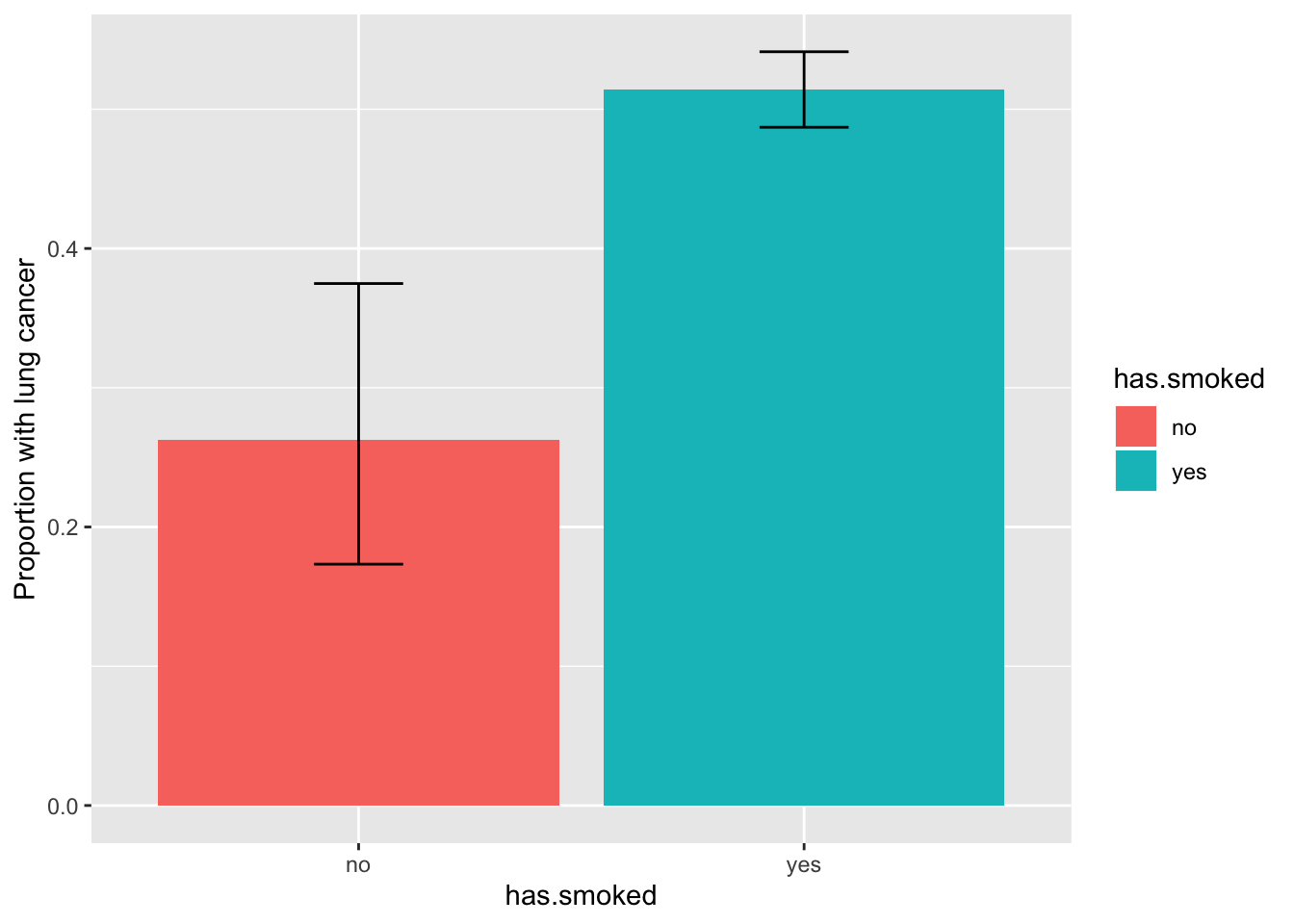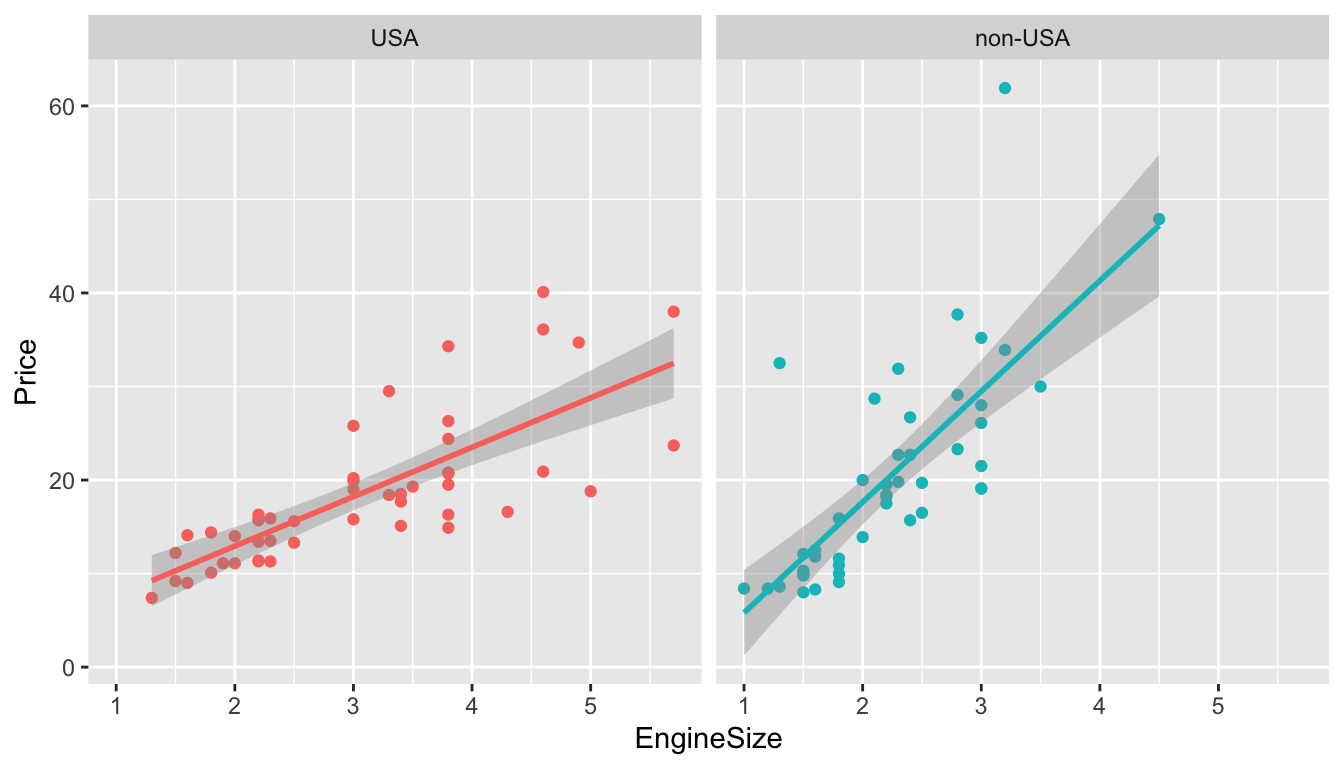##### Remember to change the `author:` field on this Rmd file to your own name.

``library(tidyverse)``
``## ── Attaching packages ─────────────────────────────────────────────────── tidyverse 1.2.1 ──``
``````## ✔ ggplot2 3.2.1     ✔ purrr   0.3.3
## ✔ tibble  2.1.3     ✔ dplyr   0.8.3
## ✔ tidyr   1.0.0     ✔ stringr 1.4.0
## ✔ readr   1.3.1     ✔ forcats 0.4.0``````
``````## ── Conflicts ────────────────────────────────────────────────────── tidyverse_conflicts() ──
``Cars93 <- as_tibble(MASS::Cars93)``

### Testing 2 x 2 tables

Doll and Hill’s 1950 article studying the association between smoking and lung cancer contains one of the most important 2 x 2 tables in history.

Here’s their data:

``````smoking <- as.table(rbind(c(688, 650), c(21, 59)))
dimnames(smoking) <- list(has.smoked = c("yes", "no"),
lung.cancer = c("yes","no"))
smoking``````
``````##           lung.cancer
## has.smoked yes  no
##        yes 688 650
##        no   21  59``````

(a) Use `fisher.test()` to test if there’s an association between smoking and lung cancer.

``````smoking.fisher.test <- fisher.test(smoking)
smoking.fisher.test``````
``````##
##  Fisher's Exact Test for Count Data
##
## data:  smoking
## p-value = 1.476e-05
## alternative hypothesis: true odds ratio is not equal to 1
## 95 percent confidence interval:
##  1.755611 5.210711
## sample estimates:
## odds ratio
##   2.971634``````

(b) What is the odds ratio? Interpret this quantity.

``smoking.fisher.test\$estimate``
``````## odds ratio
##   2.971634``````

This says that the odds of having lung cancer are 2.97 times higher among smokers than non-smokers.

(c) Are your findings statistically significant?

``smoking.fisher.test\$p.value``
``##  1.476303e-05``

The findings are highly statistically significant.

### Plotting error bars

Using Doll and Hill’s smoking data and, construct a bar graph with accompanying error bars showing the proportion of study participants with lung cancer in each smoking status group.

To succeed in this exercise, you’ll want to follow along careful with the lecture notes. Please read the section titled “Plotting the table values with confidence”.

``````# Reshape the count table into a tibble:
smoking.tbl <- as_tibble(smoking)

# Add a column showing total count in each (smoking status) group
smoking.tbl <- smoking.tbl %>%
group_by(has.smoked) %>%
mutate(total = sum(n)) %>%
filter(lung.cancer == "yes")  # Retain only the lung.cancer = yes rows

smoking.toplot <- smoking.tbl %>%
group_by(has.smoked) %>%
summarize(prop = n / total,
lower = prop.test(n, total)\$conf.int,
upper = prop.test(n, total)\$conf.int)

# Here's our summary table
smoking.toplot``````
``````## # A tibble: 2 x 4
##   has.smoked  prop lower upper
##   <chr>      <dbl> <dbl> <dbl>
## 1 no         0.262 0.173 0.375
## 2 yes        0.514 0.487 0.541``````
``````# Plotting routine
smoking.toplot %>%
ggplot(aes(x = has.smoked, fill = has.smoked, y = prop)) +
geom_bar(position="dodge", stat="identity") +
geom_errorbar(aes(ymin=lower, ymax=upper),
width=.2,                    # Width of the error bars
position=position_dodge(0.9)) +
ylab("Proportion with lung cancer")``````### ANOVA with birthwt data

Let’s form our favourite birthwt data set.

``````# Import data, rename variables, and recode factors all in one set of piped
# commands
birthwt <-  as_tibble(MASS::birthwt)  %>%
rename(birthwt.below.2500 = low,
mother.age = age,
mother.weight = lwt,
mother.smokes = smoke,
previous.prem.labor = ptl,
hypertension = ht,
uterine.irr = ui,
physician.visits = ftv,
birthwt.grams = bwt) %>%
mutate(race = recode_factor(race, `1` = "white", `2` = "black", `3` = "other")) %>%
mutate_at(c("mother.smokes", "hypertension", "uterine.irr", "birthwt.below.2500"),
~ recode_factor(.x, `0` = "no", `1` = "yes"))``````

(a) Create a new factor that categorizes the number of physician visits into four levels: 0, 1, 2, 3 or more.

``````birthwt <- birthwt %>%
mutate(physician.visits.binned = recode_factor(physician.visits,
`0` = "0",
`1` = "1",
`2` = "2",
.default = "3 or more"
))``````

Hint: One way of doing this is with `recode` by specifying `.default = "3 or more"`. Have a look at the help file for `recode` to learn more.

(b) Run an ANOVA to determine whether the average birth weight varies across number of physician visits. Interpret the results.

``summary(aov(birthwt.grams ~ physician.visits.binned, data = birthwt))``
``````##                          Df   Sum Sq Mean Sq F value Pr(>F)
## physician.visits.binned   3  2259057  753019   1.426  0.237
## Residuals               185 97710599  528165``````

We find that there is no statistically significant variation in average birthweigh across different levels of the number of first trimester physician visits.

### Linear regression with Cars93 data

Below is figure showing how Price varies with EngineSize in the Cars93, with accompanying regression lines. There are two plots, one for USA cars, and one for non-USA cars.

``````qplot(data = Cars93, x = EngineSize, y = Price, colour = Origin) +
facet_wrap("Origin") +
stat_smooth(method = "lm") +
theme(legend.position="none")``````(a) Use the `lm()` function to regress Price on EngineSize and Origin

``````cars.lm <- lm(Price ~ EngineSize + Origin, data = Cars93)

summary(cars.lm)``````
``````##
## Call:
## lm(formula = Price ~ EngineSize + Origin, data = Cars93)
##
## Residuals:
##     Min      1Q  Median      3Q     Max
## -13.474  -4.362  -1.051   2.743  34.626
##
## Coefficients:
##               Estimate Std. Error t value Pr(>|t|)
## (Intercept)    -3.0892     2.5419  -1.215    0.227
## EngineSize      7.0637     0.7617   9.274 9.24e-15 ***
## Originnon-USA   7.7596     1.5725   4.935 3.66e-06 ***
## ---
## Signif. codes:  0 '***' 0.001 '**' 0.01 '*' 0.05 '.' 0.1 ' ' 1
##
## Residual standard error: 6.948 on 90 degrees of freedom
## Multiple R-squared:  0.4939, Adjusted R-squared:  0.4826
## F-statistic: 43.91 on 2 and 90 DF,  p-value: 4.925e-14``````

(b) Run `plot()` on your `lm` object. Do you see any problems?

``````par(mfrow = c(2,2))
plot(cars.lm)``````